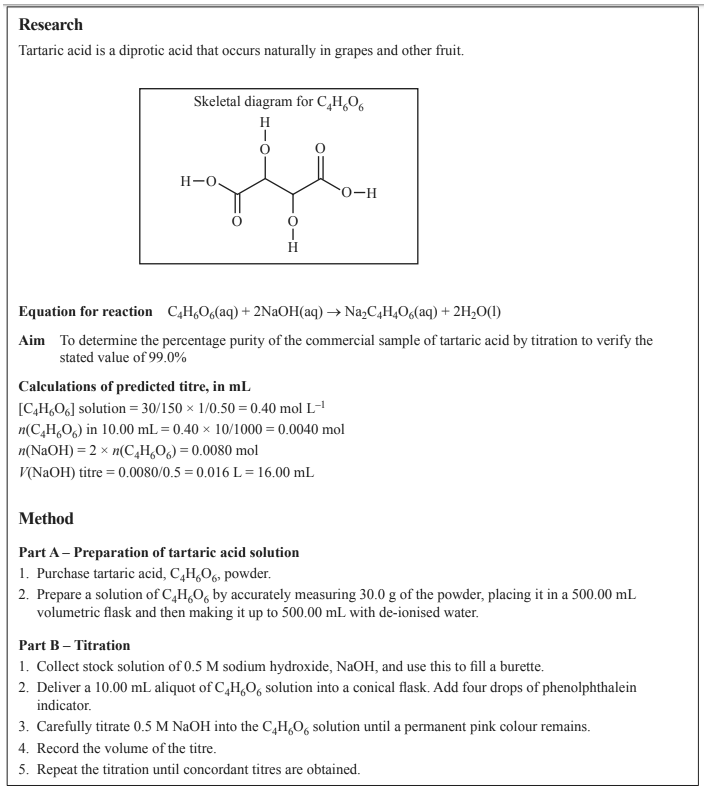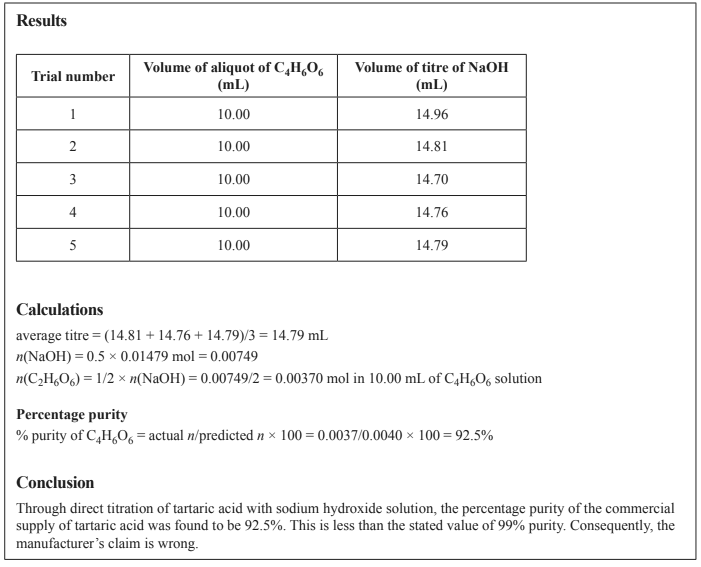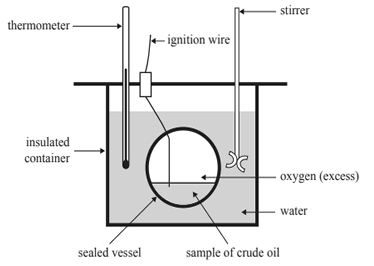Experimental technique (2017 VCE) A group of students designed and carried out an experiment to investigate if tartaric acid, C4H6O6 , that was bought commercially is 99% pure, as claimed by the manufacturer. The experiment involved titrating C4H6O6 with sodium hydroxide, NaOH, solution, calculating the percentage purity of C4H6O6 and comparing the experimental value to the manufacturer’s stated value. Part of the report submitted by one of the students is shown belowa. Name the independent variable in this experiment. b. Identify a controlled variable in this experiment and state why it is important for this variable to be controlled. Solution.c. Is the value the student used for the average titre in the ‘Calculations’ section, above, appropriate? Explain your reasoning. d. Consider the method undertaken by the student in this experiment to determine the percentage purity of C4H6O6 powder. Identify how specific steps in the method affect the accuracy and reliability of the data. Solution e. Identify a limitation of the student’s conclusion. How could this limitation be addressed? Solutionf. The material safety data sheet (MSDS) for C4H6O6 powder includes the following statement: ‘Warning! This product causes eye, skin and respiratory tract irritation.’ Apart from a laboratory coat, what personal protective equipment (PPE) should be used by the students in each of the following situations? • Preparing the C4H6O6 solution • Conducting the titration Solution2) The heat of combustion of a sample of crude oil is to be determined using a bomb calorimeter. All of the students in a class are given the same method to follow. The apparatus used by the students is shown below.For this experiment, the students could maximise A. precision by using a digital thermometer ± 0.2 °C. B. validity by calculating the heat of combustion per mole. C. accuracy by taking samples from three different sources. D. uncertainty by having all students closely follow the same experimental procedure Solution